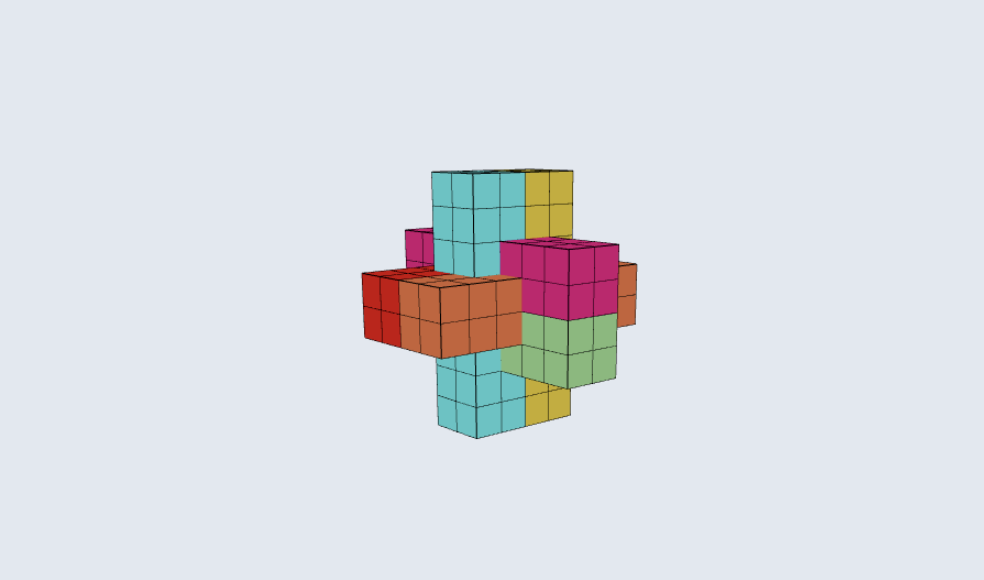# Wood Puzzle Solver

A Javascript program that solves a wood puzzle using a branch-and-bound algorithm.Javascript
Three-JS

### Intro

My roommate has been trying to solve a puzzle for the last seven years. The puzzle consists of six wooden pieces that come together to form a 3D plus sign. We talked about his attempts to solve the puzzle so far when I jokingly put forth the idea of writing a program to solve it.
Keep reading to see how I solved this problem, or jump to the mind-blowing 3D rendering of the solution!

### Step 1: Conceptual Solution

I first brainstormed different ways to solve the problem. Intuitively, I thought it would be much easier to solve the puzzle backwards, like a maze. In summary, given a solved puzzle, find the series of moves that result in each block being removed from the puzzle.
Programmatically, this was my approach.
• Create all permutations of the six pieces assembled into the final shape. We'll call these the starting shapes.
• Eliminate any starting shapes where there is overlap amongst the pieces.
• For each remaining starting shape, recursively try to move some subset of pieces one unit in any direction using branch and bound.
• Eliminate any branch if there are no possible moves.

### Step 1: Modeling

The first step was to model the wooden pieces. After looking at each block, it was clear that each block was comprised of smaller, standardized cubes. Therefore, I could represent each block as an array of cubes, each cube living at a particular coordinate in 3D space. A coordinate in 3D space can be represented as an array of the form [x,y,z]. Create an array of these coordinate arrays, and you have a block.
Below you can see a 3D visualization of the six pieces using the aforementioned modeling method.

### Step 2: Creating Starting Shapes

Since I planned to solve the puzzle backwards, the program had to start by assembling the six pieces into the final shape. However, there are many permutations to consider:
• Each of the six pieces can be placed in any of the six starting positions. That means there are six factorial permutations or 720 permutations.
• For each starting position, each piece can be in one of eight unique orientations. To be precise, in a given position, each piece can be spun four times around its long axis and placed either forward or backward. Therefore, there are 8^6 permutations for orienting each piece within a given shape, equaling 262,144 permutations.
• Therefore, there are 262,144 * 720 == 188,743,680 initial starting shapes!
Below, I show a rendering of all starting shapes where all pieces are in the first orientation.
Permutation 1 / 0

### Step 3: Eliminate Invalid Starting Shapes

In the previous step, the branch and bound algorithm created 188 million branches to evaluate. This is a lot for single-threaded JavaScript to handle, so now we start to bound.
How do we do that? We remove any starting shapes that are invalid. A starting shape is invalid if any pieces occupy the same space in 3D space. Since each piece is comprised of uniform cubes, we simply ensure that all cubes in all pieces are unique. Here you can see a visualization of when two pieces start to overlap:

### Step 4: Recursively Move Pieces

Now that we only have valid starting shapes, we can begin to move pieces. This is the real meat and potatoes of the solver.
The solver iterates through all permutations of all subsets of pieces and then recursively tries to move that subset of pieces one unit in any of the six valid directions (+x, -x, +y, -y, +z, -z). If there are any overlaps in the pieces, that branch is pruned, and the solver continues.

### Step 5: Extract All Pieces

What's the goal of the solver? To continuously move the pieces until it can remove the pieces from the puzzle altogether, one by one.
To achieve this, in every recursive call, before moving the subset of pieces 1 unit in a direction, the solver checks if any pieces can be removed from the puzzle. How? For each piece, the solver checks if that piece can be moved ten units in any direction sequentially without overlapping with another piece. If it can do that, then that piece can be removed from the puzzle, and the solver no longer has to consider it in future recursive calls.
Once the last piece is removed, the puzzle is complete! We just have to play back the sequence of piece movements in reverse to see how the puzzle is assembled.